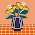Bangalore : We need to print a pyramid using * like this
*
***
*****
*******

So we need to get input from user, through console how many row he want to display..
so here is our code

In main method we will get input from user and pass that values into our print method.
so there we have 3 for loops.
first for loop for knowing which row you want to print.
second for loop for printing the spaces
third for loop for printing the *'s
just go through the source code if you have any doubts.
so we will get the result like this on Ctrl+F5 button click

using System;
using System.Collections.Generic;
using System.Linq;
using System.Text;

namespace Stars
{
class Program
{
public static void print(int n)
{

for (int i = 1; i <= n; i++)
{

for (int j = i; j <= n; j++)
{
Console.Write("  ");

}

for (int k = 1; k <= 2 * i - 1; k++)
{
Console.Write("*" + " ");
}
Console.WriteLine();
}

}

static void Main(string[] args)
{

Console.WriteLine("Enter the number of rows");
int r=Convert.ToInt32(Console.ReadLine());
print(r);
}
}
}

/* androidsharp */

#### 1 comment:

1.thank u for giving this information